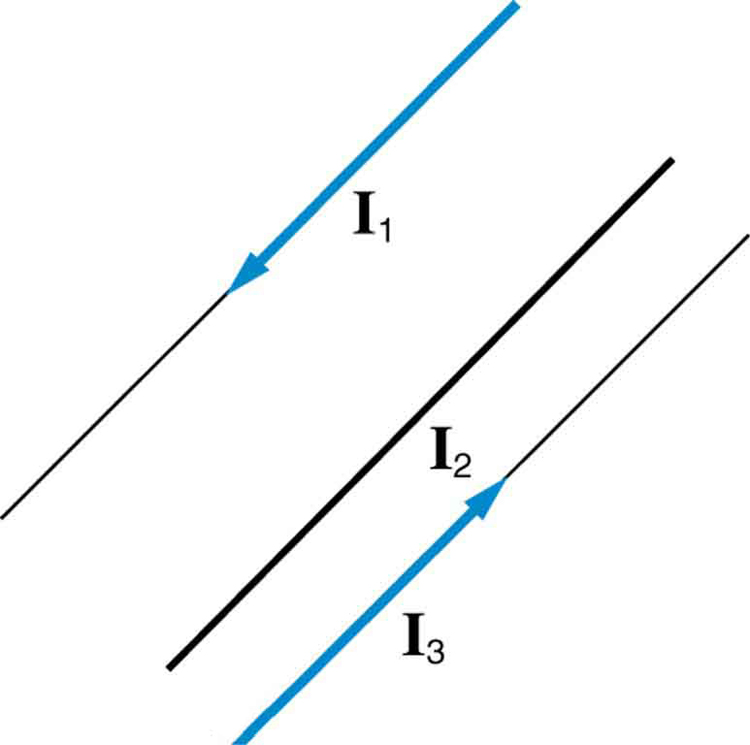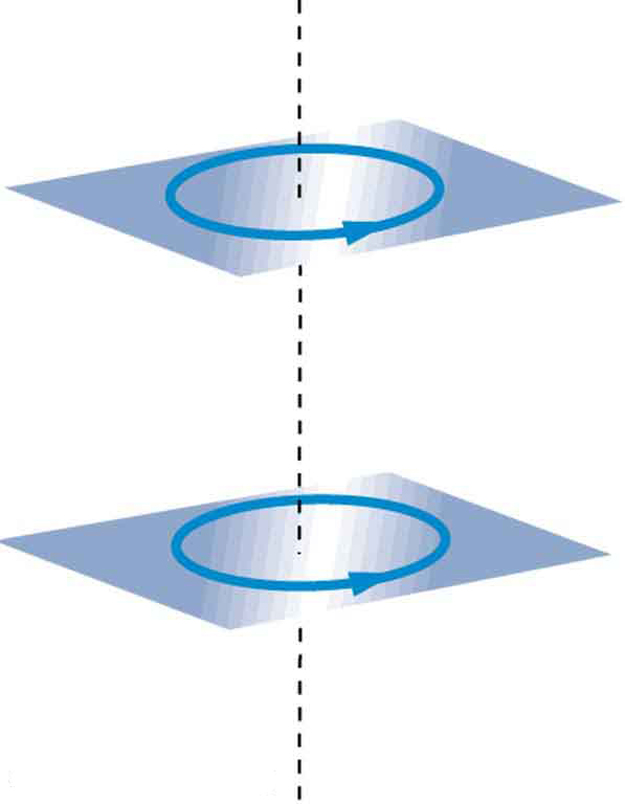# 22.10 Magnetic force between two parallel conductors  (Page 2/2)

 Page 2 / 2

Since ${\mu }_{0}$ is exactly $4\pi ×{\text{10}}^{-7}\phantom{\rule{0.25em}{0ex}}T\cdot \text{m/A}$ by definition, and because $\text{1 T}=\text{1 N/}\left(A\cdot m\right)$ , the force per meter is exactly $2×{\text{10}}^{-7}\phantom{\rule{0.25em}{0ex}}\text{N/m}$ . This is the basis of the operational definition of the ampere.

## The ampere

The official definition of the ampere is:

One ampere of current through each of two parallel conductors of infinite length, separated by one meter in empty space free of other magnetic fields, causes a force of exactly $2×{\text{10}}^{-7}\phantom{\rule{0.25em}{0ex}}\text{N/m}$ on each conductor.

Infinite-length straight wires are impractical and so, in practice, a current balance is constructed with coils of wire separated by a few centimeters. Force is measured to determine current. This also provides us with a method for measuring the coulomb. We measure the charge that flows for a current of one ampere in one second. That is, $1 C=1 A\cdot s$ . For both the ampere and the coulomb, the method of measuring force between conductors is the most accurate in practice.

## Section summary

• The force between two parallel currents ${I}_{1}$ and ${I}_{2}$ , separated by a distance $r$ , has a magnitude per unit length given by
$\frac{F}{l}=\frac{{\mu }_{0}{I}_{1}{I}_{2}}{2\mathrm{\pi r}}.$
• The force is attractive if the currents are in the same direction, repulsive if they are in opposite directions.

## Conceptual questions

Is the force attractive or repulsive between the hot and neutral lines hung from power poles? Why?

If you have three parallel wires in the same plane, as in [link] , with currents in the outer two running in opposite directions, is it possible for the middle wire to be repelled by both? Attracted by both? Explain.Three parallel coplanar wires with currents in the outer two in opposite directions.

Suppose two long straight wires run perpendicular to one another without touching. Does one exert a net force on the other? If so, what is its direction? Does one exert a net torque on the other? If so, what is its direction? Justify your responses by using the right hand rules.

Use the right hand rules to show that the force between the two loops in [link] is attractive if the currents are in the same direction and repulsive if they are in opposite directions. Is this consistent with like poles of the loops repelling and unlike poles of the loops attracting? Draw sketches to justify your answers.Two loops of wire carrying currents can exert forces and torques on one another.

If one of the loops in [link] is tilted slightly relative to the other and their currents are in the same direction, what are the directions of the torques they exert on each other? Does this imply that the poles of the bar magnet-like fields they create will line up with each other if the loops are allowed to rotate?

Electric field lines can be shielded by the Faraday cage effect. Can we have magnetic shielding? Can we have gravitational shielding?

## Problems&Exercises

(a) The hot and neutral wires supplying DC power to a light-rail commuter train carry 800 A and are separated by 75.0 cm. What is the magnitude and direction of the force between 50.0 m of these wires? (b) Discuss the practical consequences of this force, if any.

(a) 8.53 N, repulsive

(b) This forceis repulsiveand thereforethere isnever arisk thatthe twowires willtouch andshort circuit.

The force per meter between the two wires of a jumper cable being used to start a stalled car is 0.225 N/m. (a) What is the current in the wires, given they are separated by 2.00 cm? (b) Is the force attractive or repulsive?

A 2.50-m segment of wire supplying current to the motor of a submerged submarine carries 1000 A and feels a 4.00-N repulsive force from a parallel wire 5.00 cm away. What is the direction and magnitude of the current in the other wire?

400 A in the opposite direction

The wire carrying 400 A to the motor of a commuter train feels an attractive force of $4\text{.}\text{00}×{\text{10}}^{-3}\phantom{\rule{0.25em}{0ex}}\text{N/m}$ due to a parallel wire carrying 5.00 A to a headlight. (a) How far apart are the wires? (b) Are the currents in the same direction?

An AC appliance cord has its hot and neutral wires separated by 3.00 mm and carries a 5.00-A current. (a) What is the average force per meter between the wires in the cord? (b) What is the maximum force per meter between the wires? (c) Are the forces attractive or repulsive? (d) Do appliance cords need any special design features to compensate for these forces?

(a) $1\text{.}\text{67}×{\text{10}}^{-3}\phantom{\rule{0.25em}{0ex}}\text{N/m}$

(b) $3\text{.}\text{33}×{\text{10}}^{-3}\phantom{\rule{0.25em}{0ex}}\text{N/m}$

(c) Repulsive

(d) No, these are very small forces

[link] shows a long straight wire near a rectangular current loop. What is the direction and magnitude of the total force on the loop?

Find the direction and magnitude of the force that each wire experiences in [link] (a) by, using vector addition.

(a) Top wire: $2\text{.}\text{65}×{\text{10}}^{-4}\phantom{\rule{0.25em}{0ex}}\text{N/m}$ s, $\text{10}\text{.}9º$ to left of up

(b) Lower left wire: $3\text{.}\text{61}×{\text{10}}^{-4}\phantom{\rule{0.25em}{0ex}}\text{N/m}$ , $\text{13}\text{.}9º$ down from right

(c) Lower right wire: $3\text{.}\text{46}×{\text{10}}^{-4}\phantom{\rule{0.25em}{0ex}}\text{N/m}$ , $\text{30}\text{.}0º$ down from left

Find the direction and magnitude of the force that each wire experiences in [link] (b), using vector addition.

how do you calculate the 5% uncertainty of 4cm?
4cm/100×5= 0.2cm
haider
how do you calculate the 5% absolute uncertainty of a 200g mass?
= 200g±(5%)10g
haider
use the 10g as the uncertainty?
melia
haider
topic of question?
haider
the relationship between the applied force and the deflection
melia
sorry wrong question i meant the 5% uncertainty of 4cm?
melia
its 0.2 cm or 2mm
haider
thank you
melia
Hello group...
Chioma
hi
haider
well hello there
sean
hi
Noks
the meaning of phrase in physics
is the meaning of phrase in physics
Chovwe
write an expression for a plane progressive wave moving from left to right along x axis and having amplitude 0.02m, frequency of 650Hz and speed if 680ms-¹
how does a model differ from a theory
To use the vocabulary of model theory and meta-logic, a theory is a set of sentences which can be derived from a formal model using some rule of inference (usually just modus ponens). So, for example, Number Theory is the set of sentences true about numbers. But the model is a structure together wit
Jesilda
with an iterpretation.
Jesilda
what is vector quantity
Vector quality have both direction and magnitude, such as Force, displacement, acceleration and etc.
Besmellah
Is the force attractive or repulsive between the hot and neutral lines hung from power poles? Why?
what's electromagnetic induction
electromagnetic induction is a process in which conductor is put in a particular position and magnetic field keeps varying.
Lukman
wow great
Salaudeen
what is mutual induction?
je
mutual induction can be define as the current flowing in one coil that induces a voltage in an adjacent coil.
Johnson
how to undergo polarization
show that a particle moving under the influence of an attractive force mu/y³ towards the axis x. show that if it be projected from the point (0,k) with the component velocities U and V parallel to the axis of x and y, it will not strike the axis of x unless u>v²k² and distance uk²/√u-vk as origin
show that a particle moving under the influence of an attractive force mu/y^3 towards the axis x. show that if it be projected from the point (0,k) with the component velocities U and V parallel to the axis of x and y, it will not strike the axis of x unless u>v^2k^2 and distance uk^2/√u-k as origin
No idea.... Are you even sure this question exist?
Mavis
I can't even understand the question
yes it was an assignment question "^"represent raise to power pls
Gabriel
Gabriel
An engineer builds two simple pendula. Both are suspended from small wires secured to the ceiling of a room. Each pendulum hovers 2 cm above the floor. Pendulum 1 has a bob with a mass of 10kg . Pendulum 2 has a bob with a mass of 100 kg . Describe how the motion of the pendula will differ if the bobs are both displaced by 12º .
no ideas
Augstine
if u at an angle of 12 degrees their period will be same so as their velocity, that means they both move simultaneously since both both hovers at same length meaning they have the same length
Modern cars are made of materials that make them collapsible upon collision. Explain using physics concept (Force and impulse), how these car designs help with the safety of passengers.
calculate the force due to surface tension required to support a column liquid in a capillary tube 5mm. If the capillary tube is dipped into a beaker of water
find the time required for a train Half a Kilometre long to cross a bridge almost kilometre long racing at 100km/h
method of polarization
Ajayi
What is atomic number?
The number of protons in the nucleus of an atom
Deborah

#### Get Jobilize Job Search Mobile App in your pocket Now!ByByBy Angelica LitoBy OpenStaxByBy OpenStaxBy OpenStaxBy Richley CrapoBy RhodesBy OpenStaxBy Tod McGrathBy Rylee Minllic